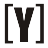# Calculator to Convert Decimal to Fraction

How to Use Enter the value of decimal and press Convert Button to get the fraction result complete with calculation.

Fraction result:
Calculation:
How to Convert Decimal to Fraction
You can converting decimal to fraction with 3 steps below:
1. Write down decimal value divided by 1
 decimal value 1

2. multiplying the numerator and denominator by 10 for every number after the decimal point. (example: if 100, use two numbers after the decimal point, if there are three then use 1000, etc.
3. simplify the fraction

Example:

Convert 0.35 to a fraction?
How to?
1. Write down 0.35 divided by 1

 0.35 1

2. multiply the numerator and denominator by 100

 0.35 = 35 1 100

3. Simplify the fraction, divided the numerator and denominator by 5 (÷5)

 0.35 = 35 = 7 1 100 20

## Decimal to Fraction Convertion Table

Decimal Fraction
0.00001 1/100000
0.0001 1/10000
0.001 1/1000
0.01 1/100
0.08333333 1/12
0.09090909 1/11
0.1 1/10
0.11111111 1/9
0.125 1/8
0.14285714 1/7
0.16666667 1/6
0.2 1/5
0.22222222 2/9
0.25 1/4
0.28571429 2/7
0.3 3/10
0.33333333 1/3
0.375 3/8
0.4 2/5
0.42857143 3/7
0.44444444 4/9
0.5 1/2
0.55555555 5/9
0.57142858 4/7
0.6 3/5
0.625 5/8
0.66666667 2/3
0.7 7/10
0.71428571 5/7
0.75 3/4
0.77777778 7/9
0.8 4/5
0.83333333 5/6
0.85714286 6/7
0.875 7/8
0.88888889 8/9
0.9 9/10
1.1 11/10
1.2 6/5
1.25 5/4
1.3 13/10
1.4 7/5
1.5 3/2
1.6 8/5
1.7 17/10
1.75 7/4
1.8 9/5
1.9 19/10
2.5 5/2YoosFuhl.com Would you like to receive notifications on latest updates? No Yes